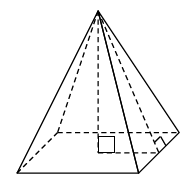### Home > GC > Chapter 11 > Lesson 11.1.3 > Problem11-36

11-36.Find the volume and surface area of a square-based right pyramid if the base edge has length $6$ units and the height of the pyramid is $4$ units. Assume the diagram at right is not to scale.

$\text{Volume}=\frac{1}{3}\left(\text{volume of the corresponding prism}\right)$

$\frac{1}{3}\left(\text{number of cubes in the bottom layer}\right)\left(\text{number of layers}\right)$

$\frac{1}{3}\left(l\right)\left(w\right)\left(h\right)$

$\frac{1}{3}\left(6^2\cdot4\right)$

$\text{Surface Area} = 4\left(\text{area of triangle}\right) + \left(\text{area of base}\right)$
You will need to find the slant height of the pyramid.

$96$ square units